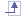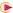# Singularity Computation

This section gives you information about the singularity computation step.## How to tune the singularity detection?

A tolerance value is used to detect singularities. Modifying this tolerance value lets you improve the singularity detection.

You can detect singularities using several environment variables:

##### Value

`ELF_Singularity_check`

This variable lets you decide to detect or not local singularities.

• `Yes`: lets you detect singularities.

• `No`: lets you ignore singularities.By default this value is equal to `Yes`.

`ELF_Singularity_constraint_type`

This variable lets you set the constraint type you want to use to correct the detected local singularities.

The possible values are:

• `mpc` (multiple point constraint): in the singular direction, the displacements are interpolated from the displacements of the neighboring nodes that are not singular.

• `spc` (single point constraint): the detected singularities are fixed in the singular direction.By default this value is equal to `mpc`.

`ELF_TranslationPivotTol`

This variable lets you fix a tolerance value (ratio between the local translational stiffness matrix pivot and the global translational stiffness matrix trace) under which the detected direction is considered as singular.By default this value is equal to `10-7`.

`ELF_RotationPivotTol`

This variable lets you fix a tolerance value (ratio between the local rotational stiffness matrix pivot and the global rotational stiffness matrix trace) under which the detected direction is considered as singular.By default this value is equal to `10-8`.

`ELF_TraceMatrixTol`

If the direction already satisfies the singularity condition in translation or in rotation, this variable lets you fix a tolerance value (ratio between full local stiffness matrix pivot and the full global stiffness matrix trace) under which the detected direction is considered as singular.By default this value is equal to `10-8`.## How does the singularity computation step work in the case of shell elements?

Because shell elements do not have rotational rigidity around their normal axis at node, a singularity is detected and fixed for this rotation in a local axis.

But when adjacent elements are not exactly in the same plane, this singularity disappears. So a tolerance is used to detect this singularity.
The tolerance used to detect this singularity has been changed for the parabolic shell elements (parabolic triangle and parabolic quadrangle) and now, more singularities are detected.

This improves the results for parabolic shell elements and especially for the parabolic triangle element. There are few impacts on the displacement results, the modal and buckling computations.## How does the singularity computation step work in the case of connection properties?

The singularity detection is done on the stiffness matrix.

If local singularities are detected on nodes impacted by kinematics relations, the local singularities are re-analyzed on the modified stiffness matrix (that is to say the stiffness matrix impacted by the kinematics relations).

In this case, additional computation steps are necessary and then:

• new steps appear in the ELFINI Solver Log file
• the performance may decrease.